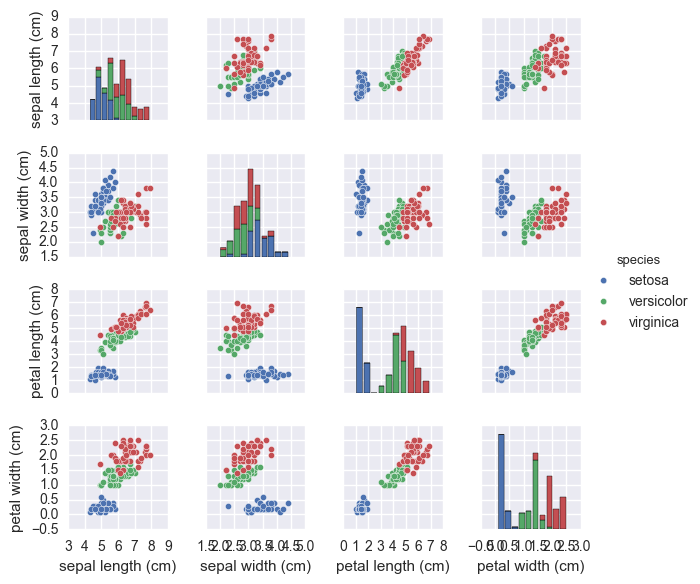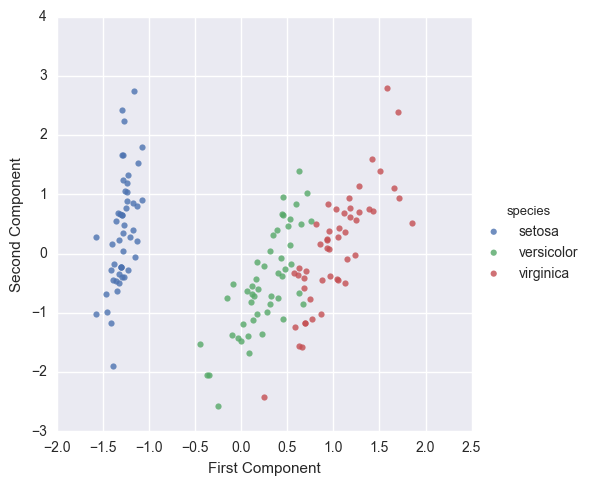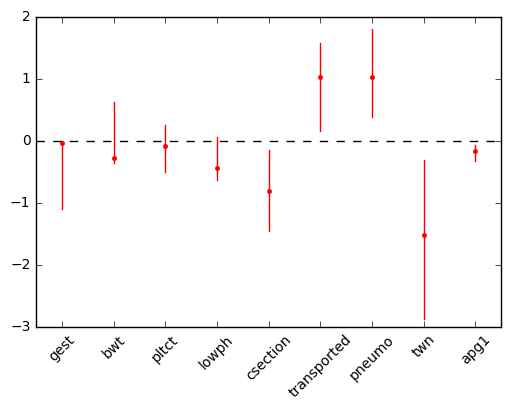# Introduction to Scikit-learn¶

The scikit-learn package is an open-source library that provides a robust set of machine learning algorithms for Python. It is built upon the core Python scientific stack (i.e. NumPy, SciPy, Cython), and has a simple, consistent API, making it useful for a wide range of statistical learning applications.## What is Machine Learning?¶

Machine Learning (ML) is about coding programs that automatically adjust their performance from exposure to information encoded in data. This learning is achieved via tunable parameters that are automatically adjusted according to performance criteria.

Machine Learning can be considered a subfield of Artificial Intelligence (AI).

There are three major classes of ML:

Supervised learning : Algorithms which learn from a training set of labeled examples (exemplars) to generalize to the set of all possible inputs. Examples of supervised learning include regression and support vector machines.

Unsupervised learning : Algorithms which learn from a training set of unlableled examples, using the features of the inputs to categorize inputs together according to some statistical criteria. Examples of unsupervised learning include k-means clustering and kernel density estimation.

Reinforcement learning : Algorithms that learn via reinforcement from a critic that provides information on the quality of a solution, but not on how to improve it. Improved solutions are achieved by iteratively exploring the solution space. We will not cover RL in this course.

## Representing Data in scikit-learn¶

Most machine learning algorithms implemented in scikit-learn expect data to be stored in a two-dimensional array or matrix. The arrays can be either numpy arrays, or in some cases scipy.sparse matrices. The size of the array is expected to be [n_samples, n_features]

• n_samples: The number of samples: each sample is an item to process (e.g. classify). A sample can be a document, a picture, a sound, a video, an astronomical object, a row in database or CSV file, or whatever you can describe with a fixed set of quantitative traits.
• n_features: The number of features or distinct traits that can be used to describe each item in a quantitative manner. Features are generally real-valued, but may be boolean or discrete-valued in some cases.

The number of features must be fixed in advance. However it can be very high dimensional (e.g. millions of features) with most of them being zeros for a given sample. This is a case where scipy.sparse matrices can be useful, in that they are much more memory-efficient than numpy arrays.

# Example: Iris morphometrics¶

One of the datasets included with scikit-learn is a set of measurements for flowers, each being a member of one of three species: Iris Setosa, Iris Versicolor or Iris Virginica.In :
from sklearn.datasets import load_iris
iris = load_iris()

In :
iris.keys()

Out:
dict_keys(['feature_names', 'target_names', 'target', 'data', 'DESCR'])
In :
n_samples, n_features = iris.data.shape
n_samples, n_features

Out:
(150, 4)
In :
iris.data

Out:
array([ 5.1,  3.5,  1.4,  0.2])

The information about the class of each sample is stored in the target attribute of the dataset:

In :
iris.target

Out:
array([0, 0, 0, 0, 0, 0, 0, 0, 0, 0, 0, 0, 0, 0, 0, 0, 0, 0, 0, 0, 0, 0, 0,
0, 0, 0, 0, 0, 0, 0, 0, 0, 0, 0, 0, 0, 0, 0, 0, 0, 0, 0, 0, 0, 0, 0,
0, 0, 0, 0, 1, 1, 1, 1, 1, 1, 1, 1, 1, 1, 1, 1, 1, 1, 1, 1, 1, 1, 1,
1, 1, 1, 1, 1, 1, 1, 1, 1, 1, 1, 1, 1, 1, 1, 1, 1, 1, 1, 1, 1, 1, 1,
1, 1, 1, 1, 1, 1, 1, 1, 2, 2, 2, 2, 2, 2, 2, 2, 2, 2, 2, 2, 2, 2, 2,
2, 2, 2, 2, 2, 2, 2, 2, 2, 2, 2, 2, 2, 2, 2, 2, 2, 2, 2, 2, 2, 2, 2,
2, 2, 2, 2, 2, 2, 2, 2, 2, 2, 2, 2])
In :
iris.target_names

Out:
array(['setosa', 'versicolor', 'virginica'],
dtype='<U10')
In :
import pandas as pd

iris_df = pd.DataFrame(iris.data, columns=iris.feature_names).assign(species=iris.target_names[iris.target])

In :
%matplotlib inline
import seaborn as sns

sns.pairplot(iris_df, hue='species', size=1.5);In :
from sklearn.decomposition import PCA

pca = PCA(n_components=2, whiten=True).fit(iris.data)
X_pca = pca.transform(iris.data)

In :
iris_df['First Component'] = X_pca[:, 0]
iris_df['Second Component'] = X_pca[:, 1]

sns.lmplot('First Component', 'Second Component',
data=iris_df,
fit_reg=False,
hue="species")

Out:
<seaborn.axisgrid.FacetGrid at 0x11080d0b8>## scikit-learn interface¶

All objects within scikit-learn share a uniform common basic API consisting of three complementary interfaces:

• estimator interface for building and ﬁtting models
• predictor interface for making predictions
• transformer interface for converting data.

The estimator interface is at the core of the library. It deﬁnes instantiation mechanisms of objects and exposes a fit method for learning a model from training data. All supervised and unsupervised learning algorithms (e.g., for classiﬁcation, regression or clustering) are oﬀered as objects implementing this interface. Machine learning tasks like feature extraction, feature selection or dimensionality reduction are also provided as estimators.

Scikit-learn strives to have a uniform interface across all methods. For example, a typical estimator follows this template:

In :
class Estimator(object):

def fit(self, X, y=None):
"""Fit model to data X (and y)"""
self.some_attribute = self.some_fitting_method(X, y)
return self

def predict(self, X_test):
"""Make prediction based on passed features"""
pred = self.make_prediction(X_test)
return pred


For a given scikit-learn estimator object named model, several methods are available. Irrespective of the type of estimator, there will be a fit method:

• model.fit : fit training data. For supervised learning applications, this accepts two arguments: the data X and the labels y (e.g. model.fit(X, y)). For unsupervised learning applications, this accepts only a single argument, the data X (e.g. model.fit(X)).

During the fitting process, the state of the estimator is stored in attributes of the estimator instance named with a trailing underscore character (_). For example, the sequence of regression trees sklearn.tree.DecisionTreeRegressor is stored in estimators_ attribute.

The predictor interface extends the notion of an estimator by adding a predict method that takes an array X_test and produces predictions based on the learned parameters of the estimator. In the case of supervised learning estimators, this method typically returns the predicted labels or values computed by the model. Some unsupervised learning estimators may also implement the predict interface, such as k-means, where the predicted values are the cluster labels.

supervised estimators are expected to have the following methods:

• model.predict : given a trained model, predict the label of a new set of data. This method accepts one argument, the new data X_new (e.g. model.predict(X_new)), and returns the learned label for each object in the array.
• model.predict_proba : For classification problems, some estimators also provide this method, which returns the probability that a new observation has each categorical label. In this case, the label with the highest probability is returned by model.predict().
• model.score : for classification or regression problems, most (all?) estimators implement a score method. Scores are between 0 and 1, with a larger score indicating a better fit.

Since it is common to modify or ﬁlter data before feeding it to a learning algorithm, some estimators in the library implement a transformer interface which deﬁnes a transform method. It takes as input some new data X_test and yields as output a transformed version. Preprocessing, feature selection, feature extraction and dimensionality reduction algorithms are all provided as transformers within the library.

unsupervised estimators will always have these methods:

• model.transform : given an unsupervised model, transform new data into the new basis. This also accepts one argument X_new, and returns the new representation of the data based on the unsupervised model.
• model.fit_transform : some estimators implement this method, which more efficiently performs a fit and a transform on the same input data.

## Regression Analysis¶

To demonstrate how scikit-learn is used, let's conduct a logistic regression analysis on a dataset for very low birth weight (VLBW) infants.

Data on 671 infants with very low (less than 1600 grams) birth weight from 1981-87 were collected at Duke University Medical Center by OShea et al. (1992). Of interest is the relationship between the outcome intra-ventricular hemorrhage and the predictors birth weight, gestational age, presence of pneumothorax, mode of delivery, single vs. multiple birth, and whether the birth occurred at Duke or at another hospital with later transfer to Duke. A secular trend in the outcome is also of interest.

The metadata for this dataset can be found here.

In :
import pandas as pd

vlbw = pd.read_csv("../data/vlbw.csv", index_col=0)

subset = vlbw[['ivh', 'gest', 'bwt', 'delivery', 'inout',
'pltct', 'lowph', 'pneumo', 'twn', 'apg1']].dropna()

# Extract response variable
y = subset.ivh.replace({'absent':0, 'possible':1, 'definite':1})

# Standardize some variables
X = subset[['gest', 'bwt', 'pltct', 'lowph']]
X0 = (X - X.mean(axis=0)) / X.std(axis=0)

# Recode some variables
X0['csection'] = subset.delivery.replace({'vaginal':0, 'abdominal':1})
X0['transported'] = subset.inout.replace({'born at Duke':0, 'transported':1})
X0[['pneumo', 'twn', 'apg1']] = subset[['pneumo', 'twn','apg1']]
X0.head()

Out:
gest bwt pltct lowph csection transported pneumo twn apg1
5 -0.381853 -0.640950 -1.756012 -1.669740 1 0 1.0 0.0 5.0
13 -0.381853 0.092756 -0.174208 -0.114754 0 0 1.0 0.0 6.0
14 -0.381853 0.370375 2.037845 1.662394 1 0 0.0 0.0 6.0
16 -1.236762 -1.255677 0.727914 0.329540 0 0 0.0 0.0 4.0
17 1.755419 1.599828 -0.124777 -0.484984 1 0 0.0 0.0 8.0

We split the data into a training set and a testing set. By default, 25% of the data is reserved for testing. This is the first of multiple ways that we will see to do this.

In :
from sklearn.model_selection import train_test_split
X_train, X_test, y_train, y_test = train_test_split(X0, y)


The LogisticRegression model in scikit-learn employs a regularization coefficient C, which defaults to 1. The amount of regularization is lower with larger values of C.

Regularization penalizes the values of regression coefficients, while smaller ones let the coefficients range widely. Scikit-learn includes two penalties: a l2 penalty which penalizes the sum of the squares of the coefficients (the default), and a l1 penalty which penalizes the sum of the absolute values.

The reason for doing regularization is to let us to include more covariates than our data might otherwise allow. We only have a few coefficients, so we will set C to a large value.

In :
from sklearn.linear_model import LogisticRegression

lrmod = LogisticRegression(C=1000)
lrmod.fit(X_train, y_train)

pred_train = lrmod.predict(X_train)
pred_test = lrmod.predict(X_test)

In :
pd.crosstab(y_train, pred_train,
rownames=["Actual"], colnames=["Predicted"])

Out:
Predicted 0 1
Actual
0 294 4
1 38 12
In :
pd.crosstab(y_test, pred_test,
rownames=["Actual"], colnames=["Predicted"])

Out:
Predicted 0 1
Actual
0 91 4
1 15 6
In :
for name, value in zip(X0.columns, lrmod.coef_):
print('{0}:\t{1:.2f}'.format(name, value))

gest:	-0.04
bwt:	-0.28
pltct:	-0.08
lowph:	-0.44
csection:	-0.80
transported:	1.03
pneumo:	1.03
twn:	-1.51
apg1:	-0.16


We can bootstrap some confidence intervals:

In :
import numpy as np

n = 1000
boot_samples = np.empty((n, len(lrmod.coef_)))

for i in np.arange(n):
boot_ind = np.random.randint(0, len(X0), len(X0))
y_i, X_i = y.values[boot_ind], X0.values[boot_ind]

lrmod_i = LogisticRegression(C=1000)
lrmod_i.fit(X_i, y_i)

boot_samples[i] = lrmod_i.coef_

In :
boot_samples.sort(axis=0)

In :
boot_se = boot_samples[[25, 975], :].T

In :
coefs = lrmod.coef_
plt.plot(coefs, 'r.')
for i in range(len(coefs)):
plt.errorbar(x=[i,i], y=boot_se[i], color='red')
plt.xlim(-0.5, 8.5)
plt.xticks(range(len(coefs)), X0.columns.values, rotation=45)
plt.axhline(0, color='k', linestyle='--')

Out:
<matplotlib.lines.Line2D at 0x112630c88>